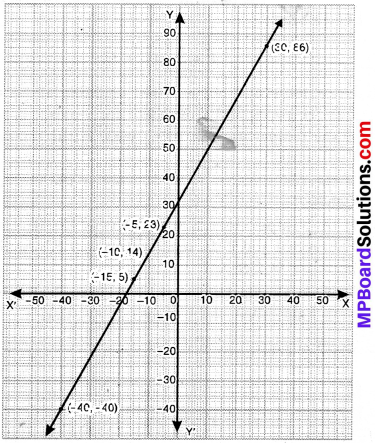## MP Board Class 9th Maths Solutions Chapter 4 Linear Equations in Two Variables Ex 4.3

Question 1.
Draw the graph of each of the following linear equations in two variables:

1. x + y = 4
2. x – y = 2
3. y = 3x
4. 3 = 2x + y

solution:
1. x + y = 4
Take x = 1
1 + y = 4
∴ y = 3

Take x = 2,
2 + y = 4
∴ y = 2

Take x = 0,
0 + y = 4
∴ y = 4

The solutions are: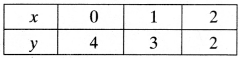The graph is shown below.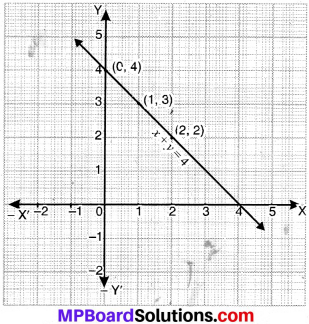2. x – y = 2
Take x = 1,
1 – y = 2
y = 2 – 1
∴ y = – 1

Take x = 2,
2 – y = 2
– y = 2 – 2
∴ y = 0

Take x = 0,
0 – y = 2
∴ y = – 2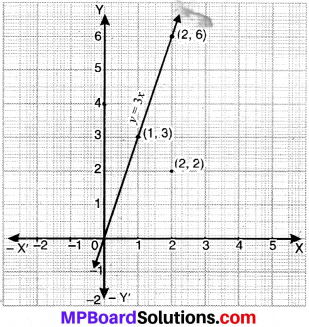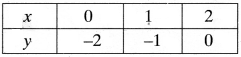3. y – 3x
Take x = 0,
y = 3 x 0 = 0

Take x = 1,
y = 3 x 1 = 3

Take x = 2,
y = 3 x 2 = 6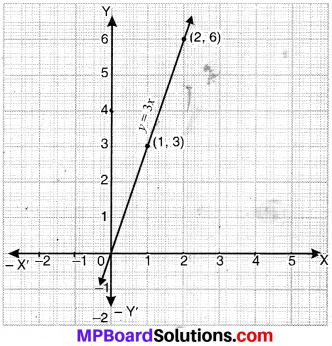4. 3 = 2x + y
Take x = 1,
3 = 2 x 1 + y
3 = 2 + y
3 – 2 = y
∴ y = 1

Take x = 0
3 = 2 x 0 + y
∴ y = 3 – 0 = 3

Take x = -1,
3 = 2 x – 1 + y
3 = – 2 + y
∴ y = 3 + 2 = 5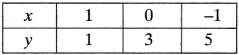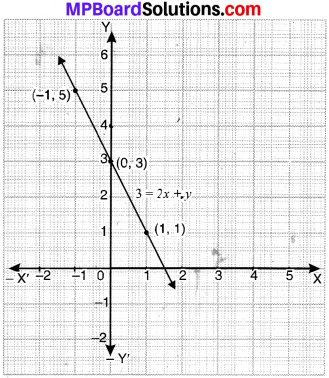Question 2.
Give the equations of two lines passing through (2, 14). How many more such lines are there and why?
Solution:
Two lines passing through point (2, 14)

1. x + y = 16
2. 2x + y – 18

Infinitely many lines can be drawn through (2, 14).Question 3.
If the point (3, 4) lies on the graph of the equation 3y = ax + 7, find the value of a.
Solution:
Putting the value of x = 3 and y = 4 in 3y = ax + 7, we get
3 x 4 = a x 3 + 7
12 = 3a + 7
3a = 12 – 7
a = $$\frac{5}{3}$$

Question 4.
The taxi fare in a city is as follows: For the first kilometer, the fare is ₹ 8 and for the subsequent distance it is ₹ 5 per km. Taking the distance covered as x km and total fare as ₹ y, write a linear equation for this information and draw its graph.
Solution:
Distance covered = x km
Total fare = ₹ y
Fare of 1st km = ₹ 8
Fare for subsequent kms = ₹ 5 per km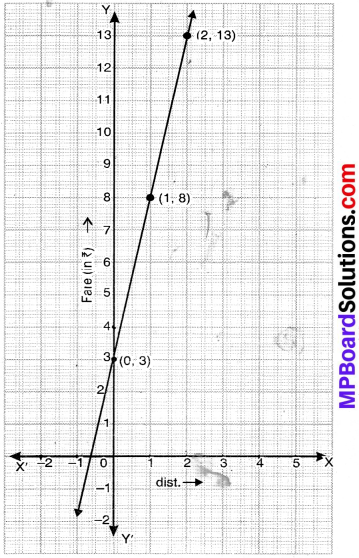According to question, y = 8 + 5 (x – 1) = 8 + 5x – 5
y = 3 + 5x
Take x = 0,
y = 3 + 5 x 0
∴ y = 3

Take x = 1,
y = 3 + 5 x 1
y = 3 + 5
∴ y = 8

Take x = 2
y = 3 + 5 x 2
∴ y = 13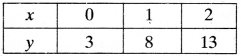Question 5.
From the choices given below, choose the equation whose graphs are given in Fig. (a) and Fig. (b)
For Fig. (a)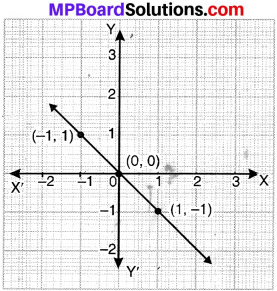1. y = x
2. x + y = 0
3. y = 2x
4. 2 + 3y = 7x

For Fig. (b)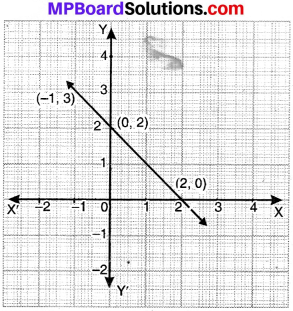1. y = x + 2
2. y = x – 2
3. y = – x + 2
4. x + 2y = 6

Solution:
1. Since (-1, 1), (0, 0) and (1, -1) satisfies the equation x + y = 0.
The equation of the graph is x + y = 0

2. Since (-1,3), (0, 2) and (2, 0) satisfies the equation y = x + 2.
The equation of the graph is y = – x + 2.Question 6.
If the work done by a body on application of a constant force is directly proportional to the distance traveled by the body, express this in the form of an equation in two variables and draw the graph of the same by taking the constant force as 5 units. Also read from the graph the work done when the distance traveled by the body is:

1. 2 units
2. 0 units.

Solution:
Let y be the work done and x be the distance covered. y ∝ x where k is the constant force.
y = kx, (Given)
k = 5
∴ y = 5x

Take x = 0
y = 5 x 0 = 0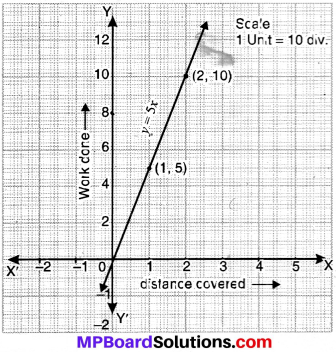Take x = 1
y = 5 x 1 = 5

Take = 2
y= 5 x 2 = 10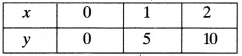When the distance travelled is –

1. x = 2 units y = 10 units
2. x = 0 units y = 0 units.

Question 7.
Yamini and Fatima, two students of class IX of a school, to gether contributed ₹ 100 towards the Prime Minister’s Relief Fund to help the earthquake victims. Write a linear equation which satisfies this data. (You may take their contributions as ₹ x and ₹ y). Draw the graph of the same.
Let the contribution of Yamini be ₹ x and that of Fatima be ₹ y.
According to question
x + y = 100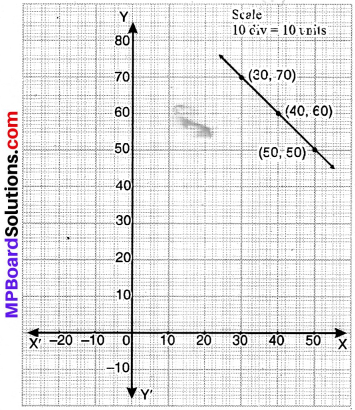Take x = 30,
30 + y = 100
y = 100 – 30
y = 70

Take = 40,
40 + y = 100
y = 100 – 40
y = 60

Take = 50,
50 + y = 100
y = 100 – 50
y = 50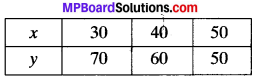Question 8.
In countries like USA and Canada, temperature is measured in Fahrenheit, whereas in countries like India, it is measured in Celsius. Here is a linear equation that converts Fahren-heit to Celsius.
F = ($$\frac{9}{5}$$) C + 32

1. Draw the graph of the linear equation above using Celsius for x – axis and Fahrenheit for y – axis.
2. If the temperature is 30°C, what is the temperature in Fahrenheit?
3. If the temperature is 95°F, what is the temperature in Celsius?
4. If the temperature is 0°C, what is the temperature in Fahrenheit and if the temperature is 0°F, what is the temperature in Celsius?
5. Is there a temperature which is numerically the same in both Fahrenheit and Celsius? If yes, find it.

1. F = $$\frac{9}{5}$$ + 32
Take C = – 5,
F = $$\frac{9}{5}$$ x (- 5) + 32
= – 9 + 32 = 23
C = – 10,
F = $$\frac{9}{5}$$ x – 10 + 32 = 14
C = – 15,
F = $$\frac{9}{5}$$ x – 15 + 32 = 5

2. From the graph, when temperature is 30°C, temperature in °F is 86°.

3. When F = 95°, C = 35°.
$$\frac{63×5}{9}$$ = 35°

4. C = 0°, F = 32°
F = 0°, C = – 17.7°

5. F = – 40°, C = – 40°